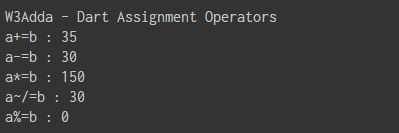# Dart Assignment operators

In this tutorial you will learn about the Dart Assignment operators and its application with practical example.

## Dart Assignment Operators

Assignment operators are used to assign value to a variable, you can assign a variable value or the result of an arithmetical expression. In many cases assignment operator can be combined with other operators to build a shorthand version of a assignment statement are known as Compound Statement. For example, instead of a = a+5 , we can write a += 5.

Dart Assignment operators
Operator Description Expression
`=` ```Assignment Operator ``` ```a=b ```
`+=` `add and assign` ```a+=b is equivalent to a=a+b ```
`-=` `subtract and assign` ```a-=b is equivalent to a=a-b ```
`*=` `multiply and assign` ```a*=b is equivalent to a=a*b ```
`/=` `divide and assign` ```a/=b is equivalent to a=a/b ```
`~/=` `divide and assign(Integer)` ```a~/=b is equivalent to a=a~/b ```
`%=` `mod and assign` ```a%=b is equivalent to a=a%b ```
`<<=` `Left shift AND assign` ```a<<=5 is equivalent to a=a<<5 ```
`>>=` `Right shift AND assign` ```a>>=5 is equivalent to a=a>>5 ```
`&=` `Bitwise AND assign` ```a&=5 is equivalent to a=a&5 ```
`^=` `Bitwise exclusive OR and assign` ```a^=5 is equivalent to a=a^5 ```
`|=` `Bitwise inclusive OR and assign` `a|=5 is equivalent to a=a|5`

Example:-

When you run the above Dart program, you will see following output.

Output:-In this tutorial we have learn about the Dart Assignment operators and its application with practical example. I hope you will like this tutorial.